# Merge Sort in Java: Algorithm & Implementation (with code)

Sorting Techniques help us to arrange the objects in a certain order. We can either use the in-built function to sort an array or different sorting techniques. But have you ever wondered which sorting technique is used in the Java in-built function "Arrays.sort(arr_name)"? Let's learn about Merge Sort in Java!

Before starting with the sorting implementation and algorithm, let's go through the basics.

## What is Merge Sort?

First, we need to revise what is Recursion. It is a process by which a function calls itself again and again until it reaches a base condition. Recursion solves the bigger problem by solving the smaller version of that problem. It solves the complex problem by breaking them into bits. The second basic topic is Divide & Conquer algorithm.

As its name suggests divide and conquer, this algorithm divides the given problem into sub-parts. It solves these sub-parts and then combines them to get the desired output.

• Divide: diving the problem into sub-problem.
• Conquer: solves the sub-problems with the help of recursion and if the sub-problem is small, it solves that directly.
• Combine: at last it combines all the solutions of the sub-problems.

Merge sort is an efficient sorting technique that uses the concept of divide and conquer algorithm in order to sort the array. Merge sort works on this ideology of diving the problem into smaller problems or subproblems to solve it efficiently.

It divides the array into 2  halves from the middle and continues doing that till it reaches a single element which means that when the middle element is the only element left. It then starts merging all the divisions and provides us with the sorted array.  To understand it more deeply let's see the illustration.

### How does Merge Sort work?

Let's learn with an example. We are given an unsorted array of size 5 and we will sort this array using merge sort.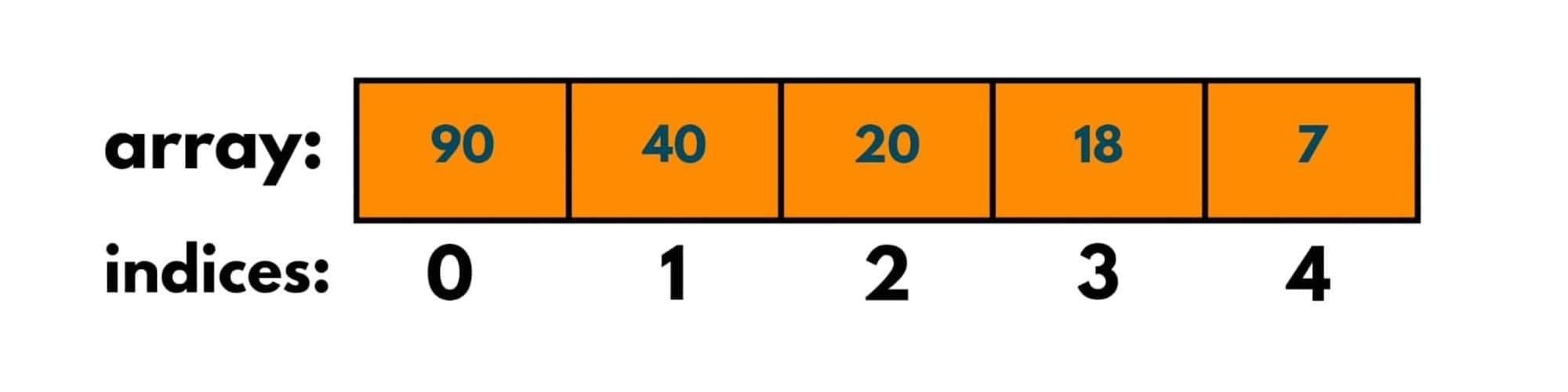So as the merge sort algorithm demands us to divide it into 2 halves, at each step we will find the mid of the array by adding the last index and first index and further dividing it by 2.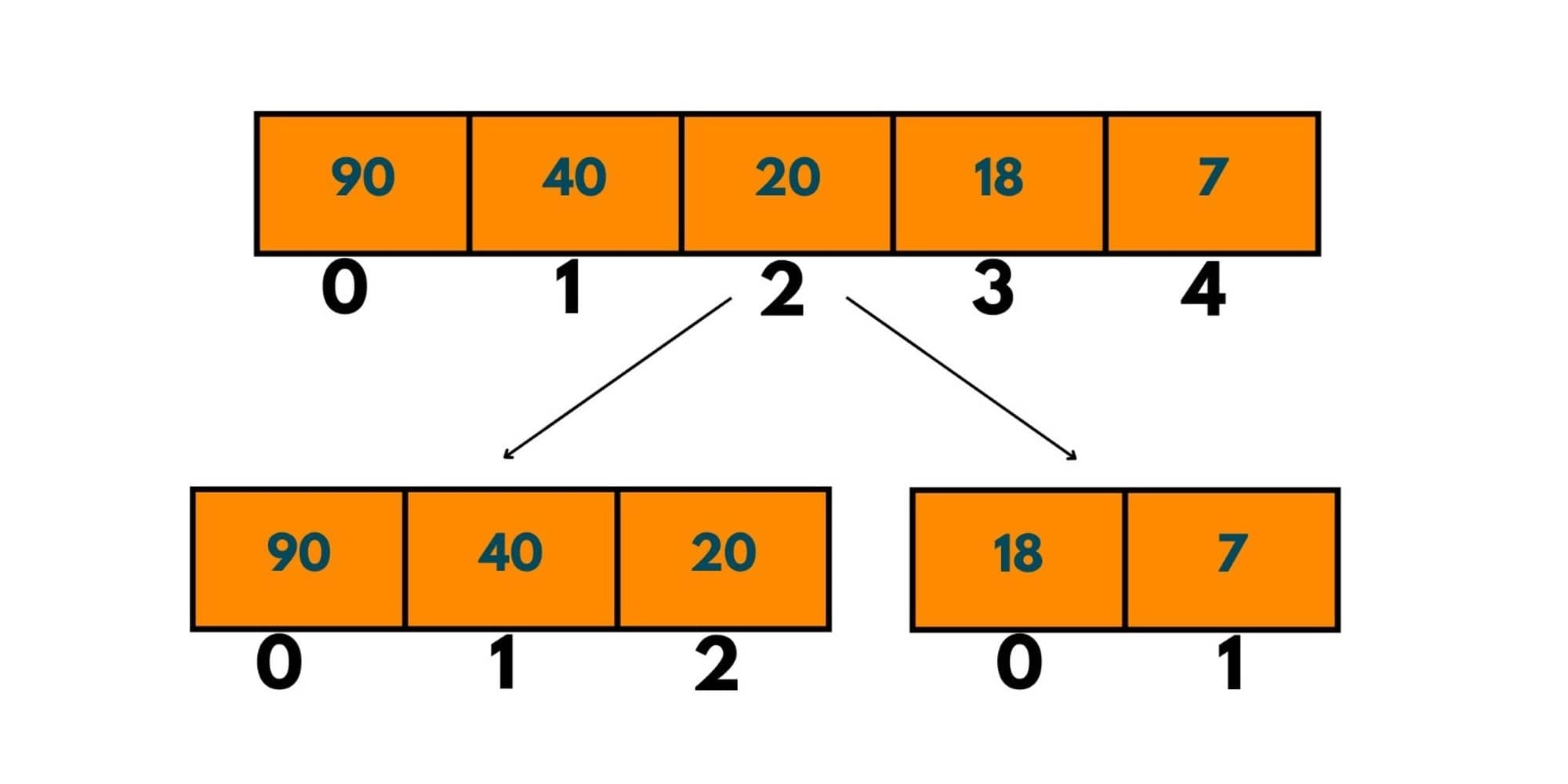The mid of the array here is { (4 +0) / 2 } which gives 2. That implies one half would contain indices [0,2] and the other half would have elements at indices [3,4]. Now we have 2 sub-arrays that would be further divided into sub-arrays with respect to their mid.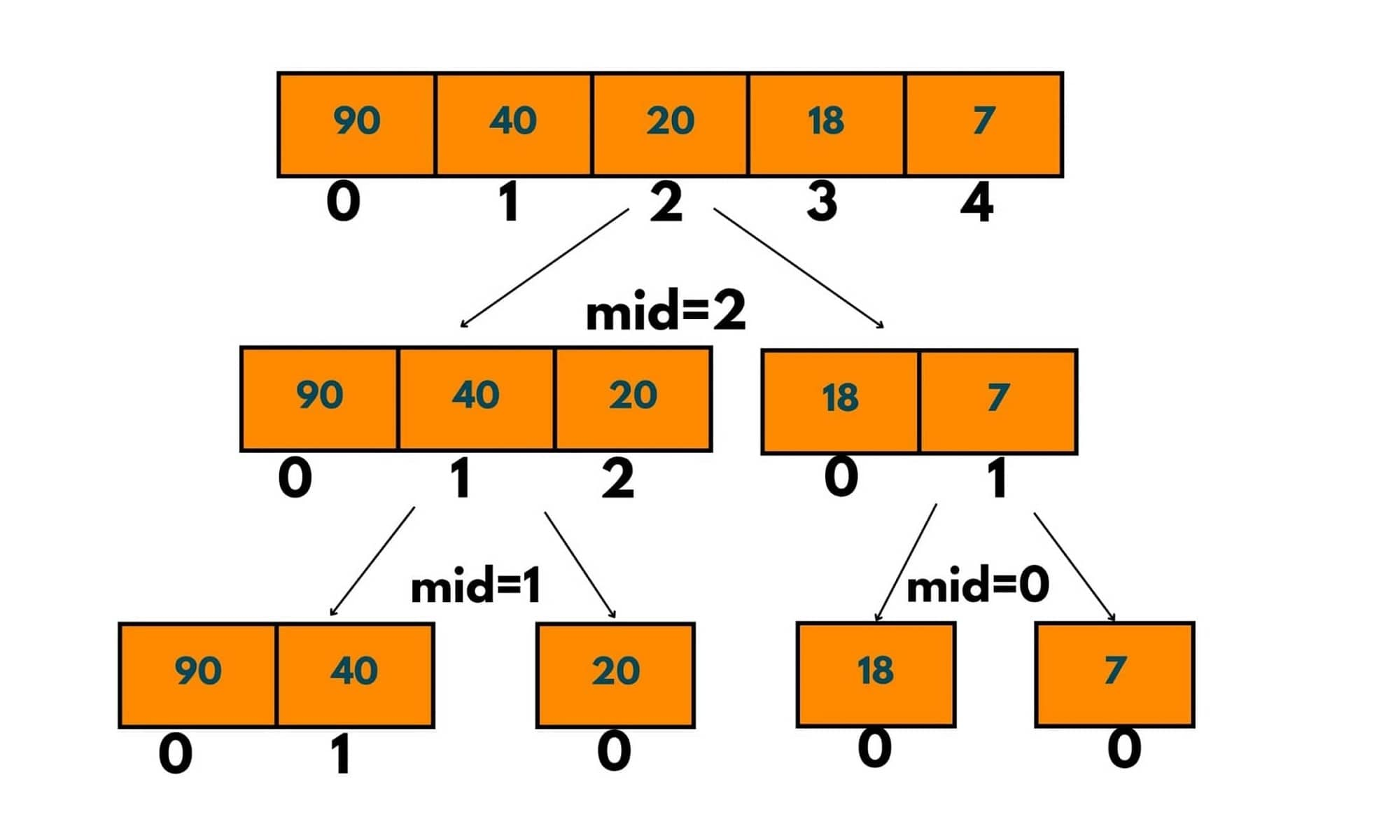Now as we can see here after further division, we reached a position where some sub-arrays have length = 1. That implies they won't be divided further. So dividing the other sub-array left.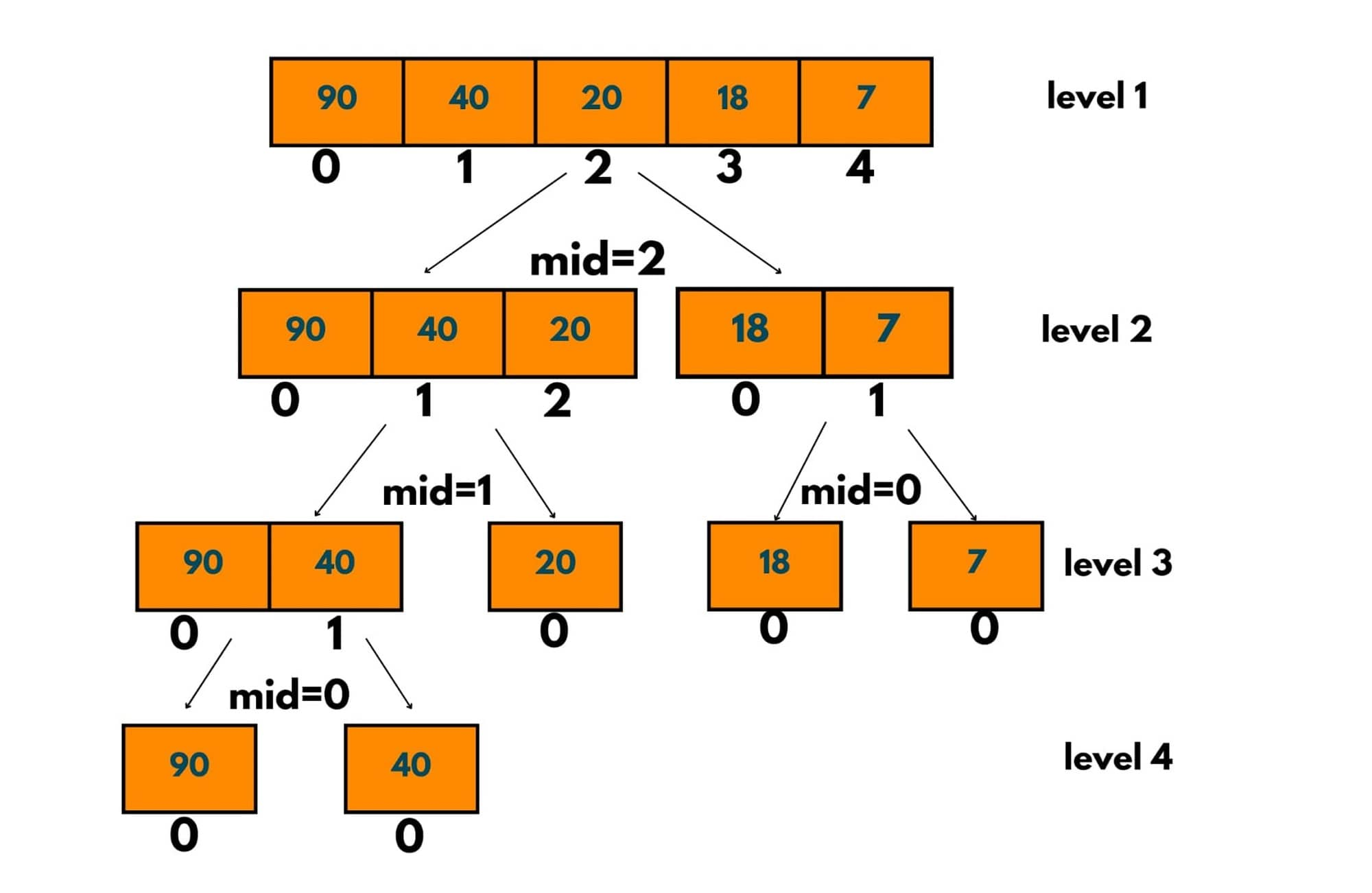Now all the sub-arrays are of length 1, i.e. the first and last index is the same, so we stop dividing the arrays here and start to merge them from the bottom, level by level.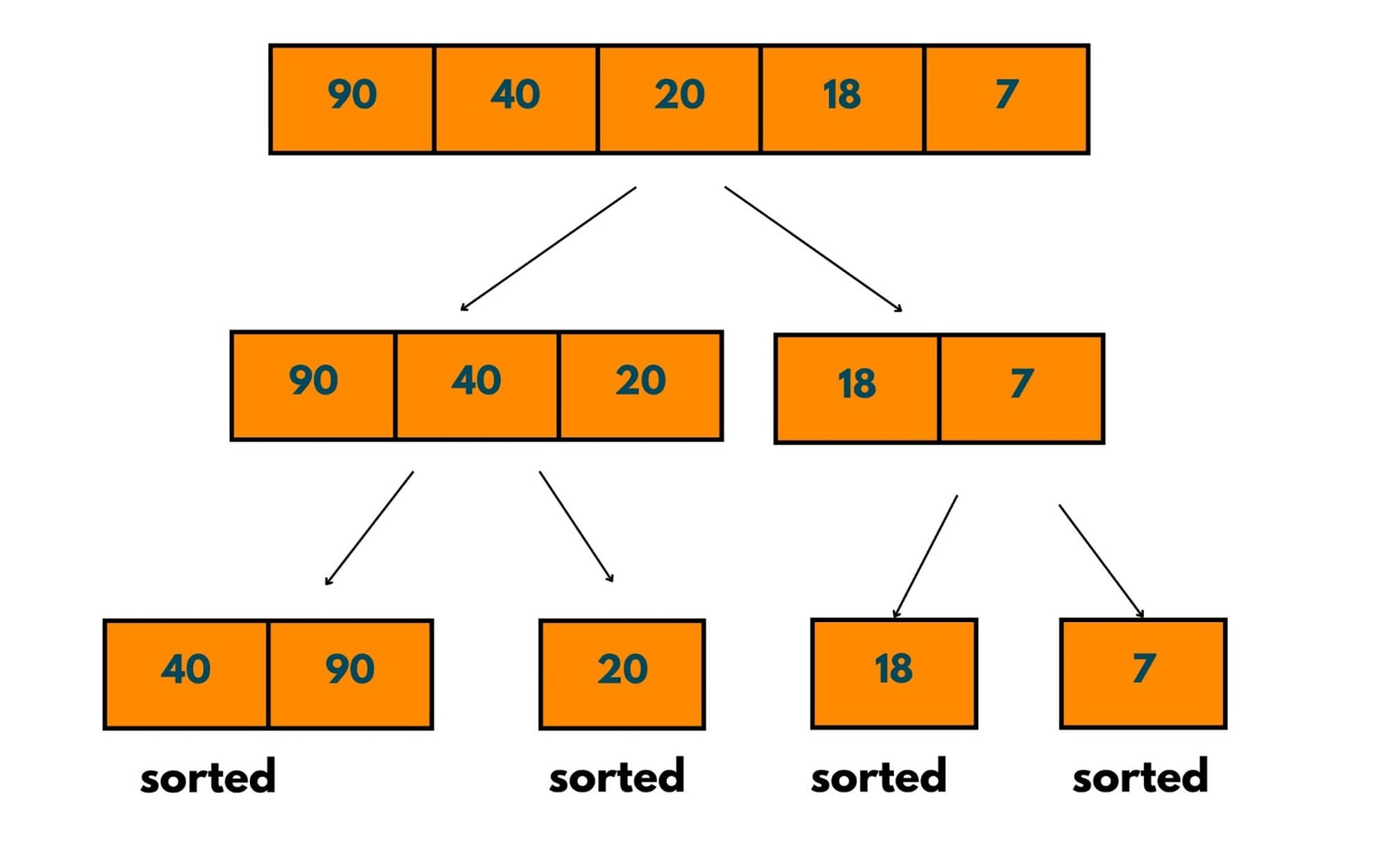This image shows the first sorting step. These single-element arrays are treated as sorted arrays and merged as how two sorted arrays are merged.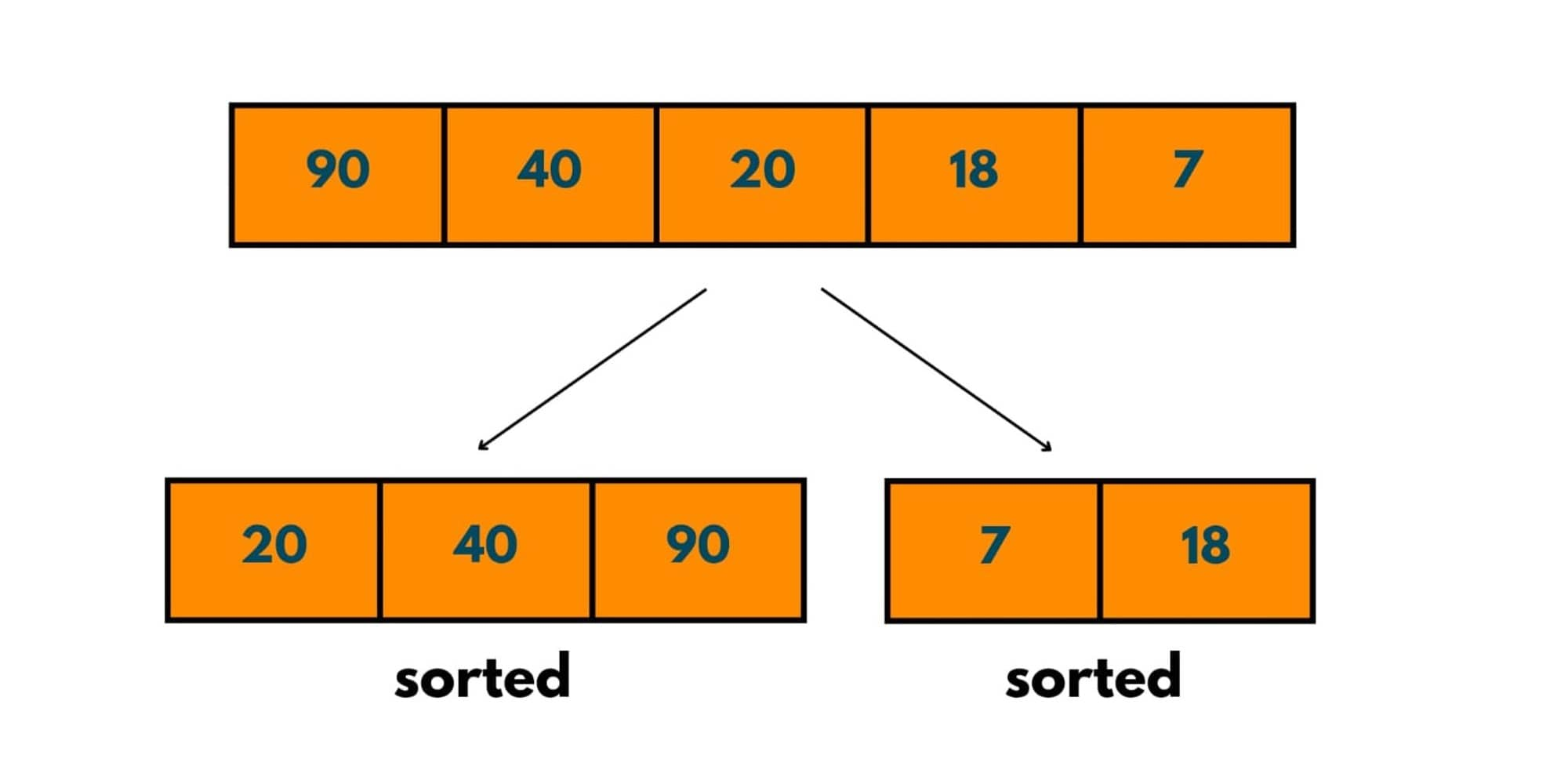Here at this. step too, we have 2 sorted arrays and we merge them using the algorithm of merging 2 sorted arrays.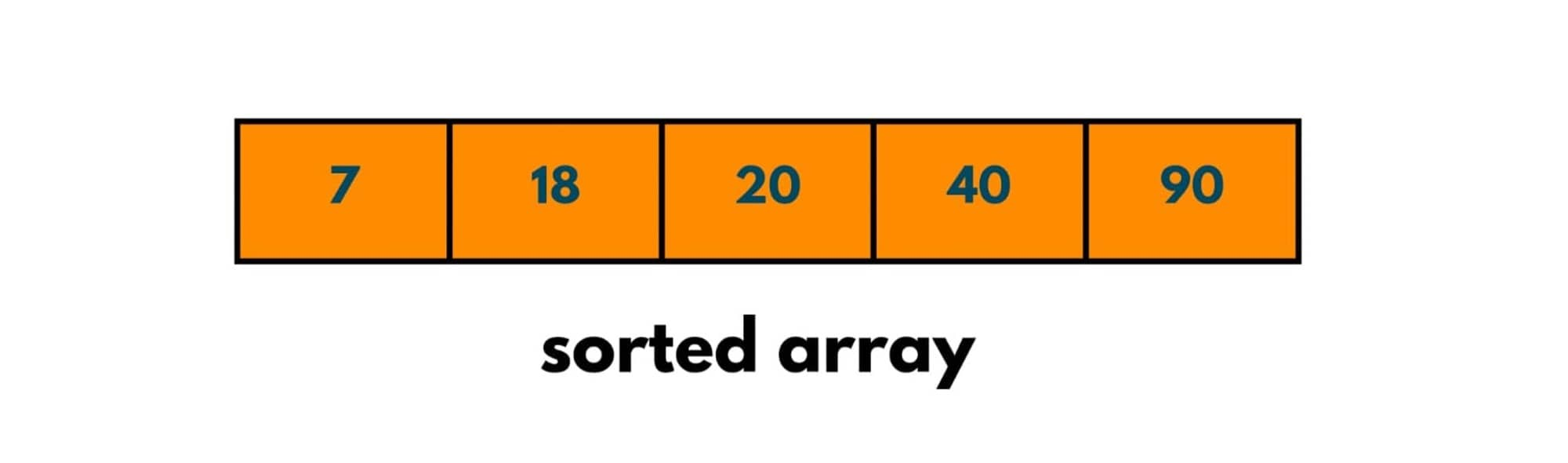This is how we proceed in the next step too and we have our array sorted.

### Merge Sort Algorithm recursive approach

Here is the simple algorithm for Merge Sort implementation using recursion:

1. We create a recursive function say mergeSort() that takes arr to be sorted as input and the first and last index of the array. Initially, we pass the first index as 0 and the last as the length of array-1.
2. We find the mid as  {(firstIndx + LastIndx) /2} to divide the array into 2 parts.
3. Now we recursively solve both parts and store the results in a new array.
4. We create an array called leftRes that would store the sorted array of the left part of the array. And we give a recursion call by passing the array and first index as 0 and the last as mid.
5. We create rightRes array to store the right sorted part of the array. And we give a recursion call by passing the array and first index as mid+1 and lastIndex as the length of array-1.
6. Then we merge both the arrays leftRes and rightRes by passing them as arguments in the function mergeTwoSortedArr.
7. When we reach a position where the last and first index is the same, i.e. now the array consists of 1 element only, we stop and put the result into a new array of size 1 and return that array.

### Merge Sort in Java

Here is the full program for Merge Sort in Java:

```import java.util.*;
public class HelloWorld {

public static int[] mergeSort(int[] arr, int firstIndx, int LastIndx) {
//base case
if(firstIndx == LastIndx) {
// putting the single element in a new array
int [] res=new int;
res=arr[firstIndx];
return res;
}
//dividing the array into 2 parts
int mid = (firstIndx + LastIndx)/2;
//sorting the left part recursively
int [] ls=mergeSort(arr,firstIndx,mid);
//sorting the right part recursively
int[] rs=mergeSort(arr,mid+1,LastIndx);
// merging the two parts
int [] ans=mergeTwoSortedArrays(ls,rs);
return ans;

}

//used for merging two sorted arrays
public static int[] mergeTwoSortedArrays(int[] arr1, int[] arr2){

int p1 = 0, p2 =0, p3 = 0;
//creating the answer array that would contain the merged sorted elements
int[] arr3 = new int[arr1.length + arr2.length];

while(p1 < arr1.length && p2 < arr2.length){
if(arr1[p1] <= arr2[p2]){
arr3[p3] = arr1[p1];
p1++;
p3++;
}else{
arr3[p3] = arr2[p2];
p2++;
p3++;
}
}

while(p1 < arr1.length){
arr3[p3] = arr1[p1];
p3++;
p1++;
}

while(p2 < arr2.length){
arr3[p3] = arr2[p2];
p3++;
p2++;
}

return arr3;
}

public static void print(int[] arr) {
for (int i = 0; i < arr.length; i++) {
System.out.print(arr[i] + " ");
}
System.out.println();
}

public static void main(String[] args)  {
int[] arr = {3,12,44,1,2,67,0};
int[] sa = mergeSort(arr,0,arr.length - 1);
System.out.print("Sorted Array -> ");
print(sa);
}

}
```

Output:

```Sorted Array -> 0 1 2 3 12 44 67
```

## How to merge two sorted Arrays?

In the working of merge sort, while going closer to the answer, we noticed that at every step we are merging 2 sorted arrays, but how is that happening? Let's learn.

Given 2 sorted arrays, say, arr1 and arr2 (can be of equal/ different lengths) and we need to combine them in a single sorted array, so in this case we take the help of the following algorithm. Here is the algorithm:

1. To reach the solution in a linear time, we take 2 pointers say p1 and p2, both pointing to the smallest element in arr1 and arr2 respectively. As both arrays are sorted p1 and p2 point to index 0.
2. We create a new array, say arr3 which will contain all the elements of arr1 and arr2 in a sorted manner, i.e. arr3 would be the merged sorted array of aa1 and arr2.
3. Therefore, the Size of arr3 = arr2.length + arr3.length. We will keep a pointer p3 that points to the 0th index of arr3 so that we can fill the element at that index.
4. Now we compare elements at p1 and p2, the smallest element of them is put into the resultant array, i.e. arr3 and we increase the pointer p3. The pointer having the smallest element is also incremented by 1.
5. We repeat step 4 till we reach a condition where either p1 = arr1.length or arr2 = arr2.length.
6. If both arrays are of unequal sizes, the array having a greater size will have some elements which have been left unexplored and not included in the resultant array, in such case we directly put the elements into arr3 till the pointer is not equal to the array's length.

Here is an example:

Given arr1 and arr2, size of arr3 = size of arr1 + size of arr2. pointers p1 = 0, p2 = 0, p3 = 0.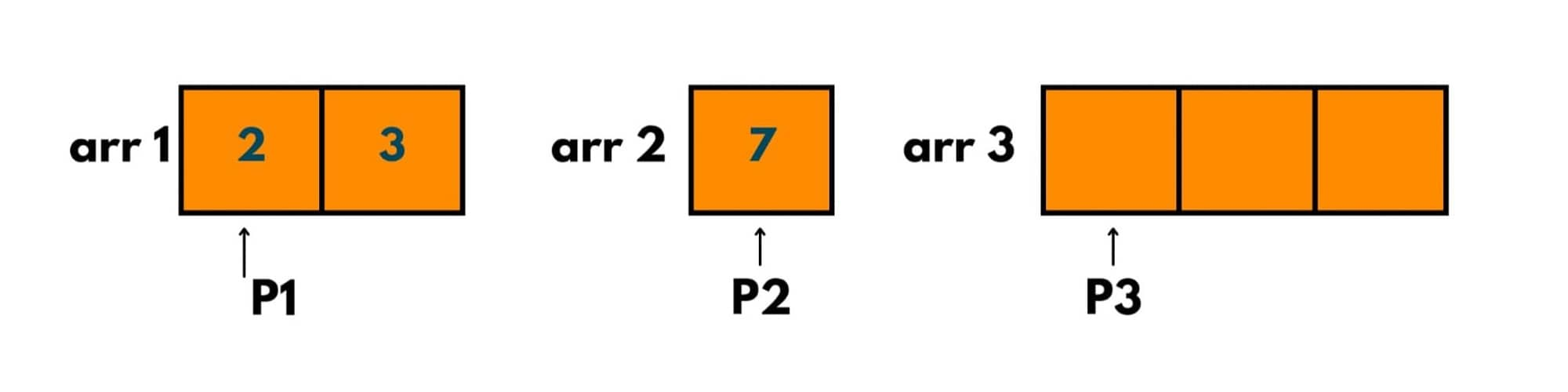As arr1[p1] < arr2[p2], we put arr3[p3] = arr1[p1]. And we increment p1 and p3 by 1.

We do the same till any of the pointers become equal to the length of the array.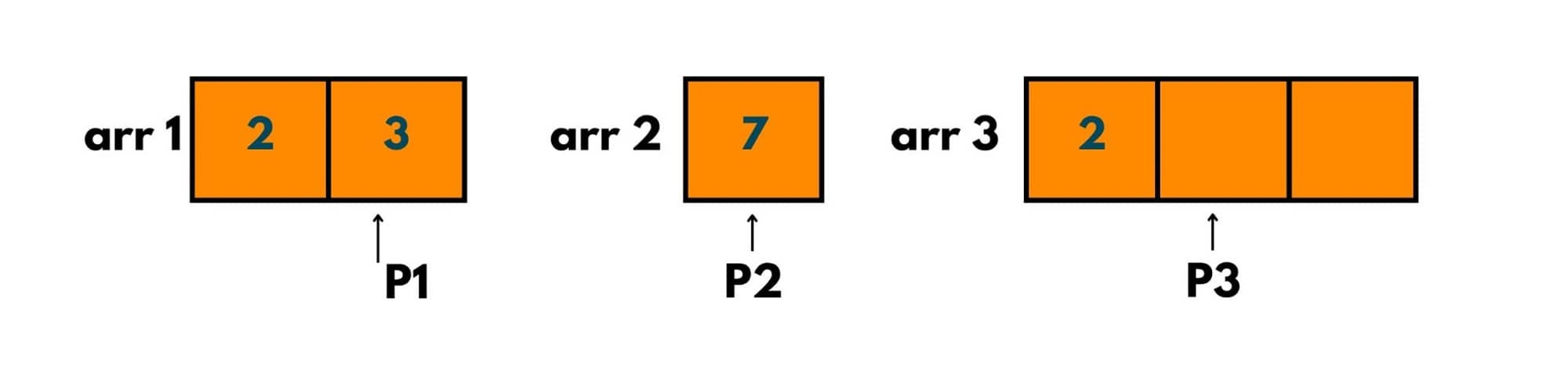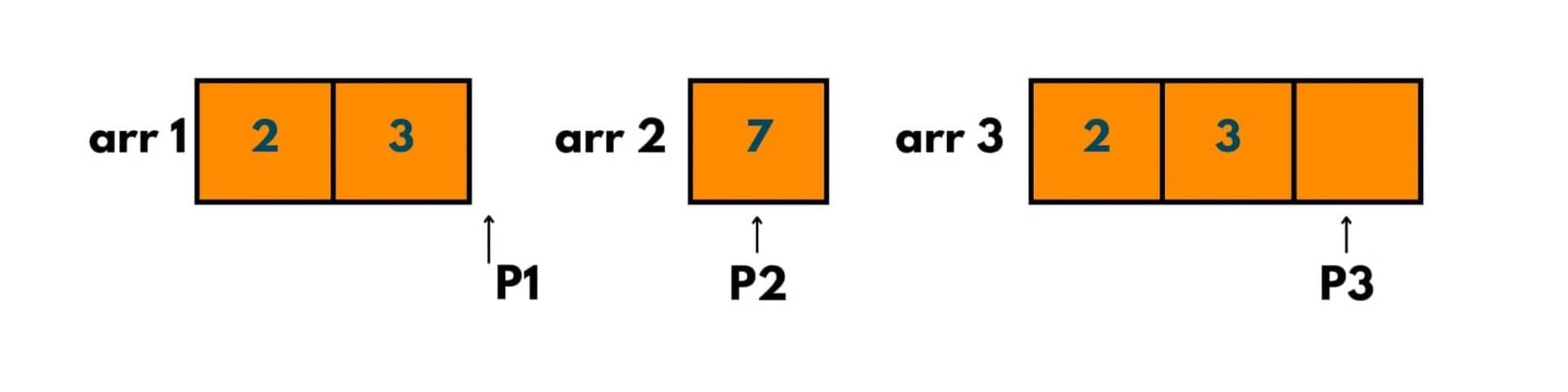As the pointer of arr1, i.e. p1 reaches the length of the array, we exit the loop. But still, we have an element that is unexplored in arr2. To put that in arr3, we now put a loop on arr2 till its pointer reaches the length of the array.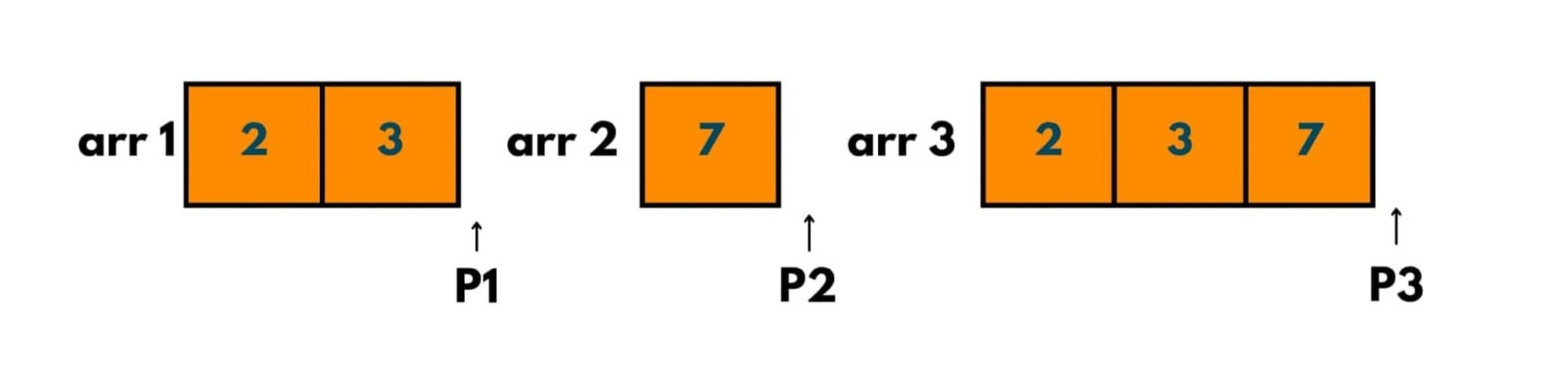and that is how we merged these arrays.

### Time & Space Complexity

As we noticed to implement the merge sort algorithm we are dividing the arrays into 2 (nearly) equal halves. So, the time complexity would be  2* T(n/2).

The time taken to merge the 2 sorted arrays is O(n) as we traversed both arrays only once. So, the recurrence relation comes out to be: T(n) = 2* T(n/2) + O(n). The solution of this recurrence relation is O(nLogn). Therefore, the time complexity of this algorithm is O(nLogn).

As 1-D arrays are used to store the array's halves, the space complexity comes out to be O(n).

### Is Merge Sort better than Quick Sort?

It depends completely on the input array or the data set that has been given.

Quick Sort has better average-case time complexity than merge sort and it uses less extra memory than merge sort. This is why it is a good option to opt for quick sorting while dealing with large data sets. But in the worst-case scenario quick sort time complexity becomes O(n^2) while merge sort has O(nLogn).

Merge Sort maintains the relative order of equal elements i.e. it is a stable sorting algorithm and it should be preferred whenever we need to maintain the relative order. n the case of LinkedList, it is the best sorting technique to implement.

### Is Merge Sort an in-place and stable algorithm?

An in-place algorithm is one in which the changes happen in the input array only and no additional memory is used except for the input array. As merge sort uses extra space of O(N) to store the subarrays is not an in-place algorithm.

A stable sorting technique is one in which the relative order of elements is maintained.  In merge sort, no relative order of equal elements is changed, hence it is a stable sorting algorithm.

Merge Sort efficiently sorts the given list in O(nlogn) time and it has a consistent running time. It also works quicker than bubble sort or insertion sort as it doesn't traverse the whole array several times.

But it is also inefficient for smaller-sized arrays and it is better to use other sorting techniques to sort an array of smaller size. Even if the array is already sorted, merge sort goes through the whole process of divide and conquer.

You can now check other popular sorting algorithms in Java as well.

## Conclusion

Overall, Merge sort is widely used to sort datasets and it is also used in Java as a built-in function called Arrays.sort() to sort an array. The wise approach of the merge sort algorithm leads to a better time complexity than any other sorting technique.

### FavTutor - 24x7 Live Coding Help from Expert Tutors!